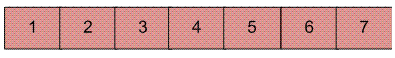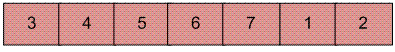# C++ Program for Block swap algorithm for array rotation

• Difficulty Level : Medium
• Last Updated : 13 Dec, 2021

Write a function rotate(ar[], d, n) that rotates arr[] of size n by d elements.Rotation of the above array by 2 will make arrayAlgorithm :

```Initialize A = arr[0..d-1] and B = arr[d..n-1]
1) Do following until size of A is equal to size of B

a)  If A is shorter, divide B into Bl and Br such that Br is of same
length as A. Swap A and Br to change ABlBr into BrBlA. Now A
is at its final place, so recur on pieces of B.

b)  If A is longer, divide A into Al and Ar such that Al is of same
length as B Swap Al and B to change AlArB into BArAl. Now B
is at its final place, so recur on pieces of A.

2)  Finally when A and B are of equal size, block swap them.```

Recursive Implementation:

## C++

 `#include ``using` `namespace` `std;`` ` `/*Prototype for utility functions */``void` `printArray(``int` `arr[], ``int` `size); ``void` `swap(``int` `arr[], ``int` `fi, ``int` `si, ``int` `d); `` ` `void` `leftRotate(``int` `arr[], ``int` `d, ``int` `n) ``{ ``    ``/* Return If number of elements to be rotated  ``    ``is zero or equal to array size */``    ``if``(d == 0 || d == n) ``        ``return``; ``         ` `    ``/*If number of elements to be rotated ``    ``is exactly half of array size */``    ``if``(n - d == d) ``    ``{ ``        ``swap(arr, 0, n - d, d); ``        ``return``; ``    ``} ``         ` `    ``/* If A is shorter*/`        `    ``if``(d < n - d) ``    ``{ ``        ``swap(arr, 0, n - d, d); ``        ``leftRotate(arr, d, n - d);     ``    ``} ``    ``else` `/* If B is shorter*/`        `    ``{ ``        ``swap(arr, 0, d, n - d); ``        ``leftRotate(arr + n - d, 2 * d - n, d); ``/*This is tricky*/``    ``} ``} `` ` `/*UTILITY FUNCTIONS*/``/* function to print an array */``void` `printArray(``int` `arr[], ``int` `size) ``{ ``    ``int` `i; ``    ``for``(i = 0; i < size; i++) ``        ``cout << arr[i] << ``" "``; ``    ``cout << endl; ``} `` ` `/*This function swaps d elements starting at index fi ``with d elements starting at index si */``void` `swap(``int` `arr[], ``int` `fi, ``int` `si, ``int` `d) ``{ ``    ``int` `i, temp; ``    ``for``(i = 0; i < d; i++) ``    ``{ ``        ``temp = arr[fi + i]; ``        ``arr[fi + i] = arr[si + i]; ``        ``arr[si + i] = temp; ``    ``} ``} `` ` `// Driver Code``int` `main() ``{ ``    ``int` `arr[] = {1, 2, 3, 4, 5, 6, 7}; ``    ``leftRotate(arr, 2, 7); ``    ``printArray(arr, 7); ``    ``return` `0; ``} `` ` `// This code is contributed by rathbhupendra`

Iterative Implementation:
Here is iterative implementation of the same algorithm. Same utility function swap() is used here.

## C++

 `// C++ code for above implementation``void` `leftRotate(``int` `arr[], ``int` `d, ``int` `n)``{``    ``int` `i, j;``    ``if` `(d == 0 || d == n)``        ``return``;``         ` `    ``i = d;``    ``j = n - d;``    ``while` `(i != j)``    ``{``        ``if` `(i < j) ``/*A is shorter*/``        ``{``            ``swap(arr, d - i, d + j - i, i);``            ``j -= i;``        ``}``        ``else` `/*B is shorter*/``        ``{``            ``swap(arr, d - i, d, j);``            ``i -= j;``        ``}``         ` `        ``// printArray(arr, 7);``    ``}``     ` `    ``/*Finally, block swap A and B*/``    ``swap(arr, d - i, d, i);``}`` ` `// This code is contributed by Shivani`

Time Complexity: O(n)
Please see following posts for other methods of array rotation:
https://www.geeksforgeeks.org/array-rotation/
https://www.geeksforgeeks.org/program-for-array-rotation-continued-reversal-algorithm/

References:
http://www.cs.bell-labs.com/cm/cs/pearls/s02b.pdf
Please write comments if you find any bug in the above programs/algorithms or want to share any additional information about the block swap algorithm.

Please refer complete article on Block swap algorithm for array rotation for more details!

My Personal Notes arrow_drop_up